Back

## Introduction To Trigonometry

### Exercise 8.1 Page: 181

1. In ∆ ABC, right-angled at B, AB = 24 cm, BC = 7 cm. Determine:

(i) sin A, cos A

(ii) sin C, cos C

Solution:

In a given triangle ABC, right angled at B = ∠B = 90°

Given: AB = 24 cm and BC = 7 cm

According to the Pythagoras Theorem,

In a right- angled triangle, the squares of the hypotenuse side is equal to the sum of the squares of the other two sides.

By applying Pythagoras theorem, we get

AC2=AB2+BC2

AC2 = (24)2+72

AC2 = (576+49)

AC2 = 625cm2

AC = √625 = 25

Therefore, AC = 25 cm

(i) To find Sin (A), Cos (A)

We know that sine (or) Sin function is the equal to the ratio of length of the opposite side to the hypotenuse side. So it becomes

Sin (A) = Opposite side /Hypotenuse = BC/AC = 7/25

Cosine or Cos function is equal to the ratio of the length of the adjacent side to the hypotenuse side and it becomes,

Cos (A) = Adjacent side/Hypotenuse = AB/AC = 24/25

(ii) To find Sin (C), Cos (C)

Sin (C) = AB/AC = 24/25

Cos (C) = BC/AC = 7/25

2. In Fig. 8.13, find tan P – cot R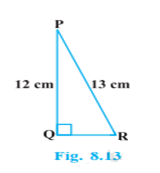Solution:

In the given triangle PQR, the given triangle is right angled at Q and the given measures are:

PR = 13cm,

PQ = 12cm

Since the given triangle is right angled triangle, to find the side QR, apply the Pythagorean theorem

According to Pythagorean theorem,

In a right- angled triangle, the squares of the hypotenuse side is equal to the sum of the squares of the other two sides.

PR2 = QR2 + PQ2

Substitute the values of PR and PQ

13= QR2+122

169 = QR2+144

Therefore, QR= 169−144

QR= 25

QR = √25 = 5

Therefore, the side QR = 5 cm

To find tan P – cot R:

According to the trigonometric ratio, the tangent function is equal to the ratio of the length of the opposite side to the adjacent sides, the value of tan (P) becomes

tan (P) Opposite side /Adjacent side = QR/PQ = 5/12

Since cot function is the reciprocal of the tan function, the ratio of cot function becomes,

Cot (R) = Adjacent side/Opposite side = QR/PQ = 5/12

Therefore,

tan (P) – cot (R) = 5/12 – 5/12 = 0

Therefore, tan(P) – cot(R) = 0

3. If sin A = 3/4, Calculate cos A and tan A.

Solution:

Let us assume a right angled triangle ABC, right angled at B

Given: Sin A = 3/4

We know that, Sin function is the equal to the ratio of length of the opposite side to the hypotenuse side.

Therefore, Sin A = Opposite side /Hypotenuse= 3/4

Let BC be 3k and AC will be 4k

where k is a positive real number.

According to the Pythagoras theorem, the squares of the hypotenuse side is equal to the sum of the squares of the other two sides of a right angle triangle and we get,

AC2=AB+ BC2

Substitute the value of AC and BC

(4k)2=AB2 + (3k)2

16k2−9k=AB2

AB2=7k2

Therefore, AB = √7k

Now, we have to find the value of cos A and tan A

We know that,

Substitute the value of AB and AC and cancel the constant k in both numerator and denominator, we get

AB/AC = √7k/4k = √7/4

Therefore, cos (A) = √7/4

Substitute the Value of BC and AB and cancel the constant k in both numerator and denominator, we get,

BC/AB = 3k/√7k = 3/√7

Therefore, tan A = 3/√7

4. Given 15 cot A = 8, find sin A and sec A.

Solution:

Let us assume a right angled triangle ABC, right angled at B

Given: 15 cot A = 8

So, Cot A = 8/15

We know that, cot function is the equal to the ratio of length of the adjacent side to the opposite side.

Therefore, cot A = Adjacent side/Opposite side = AB/BC = 8/15

Let AB be 8k and BC will be 15k

Where, k is a positive real number.

According to the Pythagoras theorem, the squares of the hypotenuse side is equal to the sum of the squares of the other two sides of a right angle triangle and we get,

AC2=AB+ BC2

Substitute the value of AB and BC

AC2= (8k)2 + (15k)2

AC2= 64k2 + 225k2

AC2= 289k2

Therefore, AC = 17k

Now, we have to find the value of sin A and sec A

We know that,

Sin (A) = Opposite side /Hypotenuse

Substitute the value of BC and AC and cancel the constant k in both numerator and denominator, we get

Sin A = BC/AC = 15k/17k = 15/17

Therefore, sin A = 15/17

Since secant or sec function is the reciprocal of the cos function which is equal to the ratio of the length of the hypotenuse side to the adjacent side.

Substitute the Value of BC and AB and cancel the constant k in both numerator and denominator, we get,

AC/AB = 17k/8k = 17/8

Therefore sec (A) = 17/8

5. Given sec θ = 13/12 Calculate all other trigonometric ratios

Solution:

We know that sec function is the reciprocal of the cos function which is equal to the ratio of the length of the hypotenuse side to the adjacent side

Let us assume a right angled triangle ABC, right angled at B

sec θ =13/12 = Hypotenuse/Adjacent side = AC/AB

Let AC be 13k and AB will be 12k

Where, k is a positive real number.

According to the Pythagoras theorem, the squares of the hypotenuse side is equal to the sum of the squares of the other two sides of a right angle triangle and we get,

AC2=AB+ BC2

Substitute the value of AB and AC

(13k)2= (12k)2 + BC2

169k2= 144k2 + BC2

169k2= 144k2 + BC2

BC2 = 169k2 – 144k2

BC2= 25k2

Therefore, BC = 5k

Now, substitute the corresponding values in all other trigonometric ratios

So,

Sin θ = Opposite Side/Hypotenuse = BC/AC = 5/13

Cos θ = Adjacent Side/Hypotenuse = AB/AC = 12/13

tan θ = Opposite Side/Adjacent Side = BC/AB = 5/12

Cosec θ = Hypotenuse/Opposite Side = AC/BC = 13/5

cot θ = Adjacent Side/Opposite Side = AB/BC = 12/5

6. If ∠A and ∠B are acute angles such that cos A = cos B, then show that ∠ A = ∠ B.

Solution:

Let us assume the triangle ABC in which CD⊥AB

Give that the angles A and B are acute angles, such that

Cos (A) = cos (B)

As per the angles taken, the cos ratio is written as

Now, interchange the terms, we get

Let take a constant value

Now consider the equation as

AC = k BC …(2)

By applying Pythagoras theorem in △CAD and △CBD we get,

CD2 = BC2 – BD… (3)

From the equations (3) and (4) we get,

Now substitute the equations (1) and (2) in (3) and (4)

K2(BC2−BD2)=(BC2−BD2) k2=1

Putting this value in equation, we obtain

AC = BC

∠A=∠B (Angles opposite to equal side are equal-isosceles triangle)

7. If cot θ = 7/8, evaluate :

(i) (1 + sin θ)(1 – sin θ)/(1+cos θ)(1-cos θ)

(ii) cot2 θ

Solution:

Let us assume a △ABC in which ∠B = 90° and ∠C = θ

Given:

cot θ = BC/AB = 7/8

Let BC = 7k and AB = 8k, where k is a positive real number

According to Pythagoras theorem in △ABC we get.

AC= AB2+BC2

AC= (8k)2+(7k)2

AC= 64k2+49k2

AC= 113k2

AC = √113 k

According to the sine and cos function ratios, it is written as

sin θ = AB/AC = Opposite Side/Hypotenuse = 8k/√113 k = 8/√113 and

cos θ = Adjacent Side/Hypotenuse = BC/AC = 7k/√113 k = 7/√113

Now apply the values of sin function and cos function: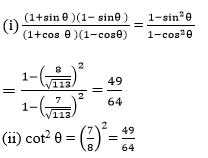8. If 3 cot A = 4, check whether (1-tanA)/(1+tan2 A) = cos2 A – sin A or not.

Solution:

Let △ABC in which ∠B=90°

We know that, cot function is the reciprocal of tan function and it is written as

cot(A) = AB/BC = 4/3

Let AB = 4k an BC =3k, where k is a positive real number.

According to the Pythagorean theorem,

AC2=AB2+BC2

AC2=(4k)2+(3k)2

AC2=16k2+9k2

AC2=25k2

AC=5k

Now, apply the values corresponding to the ratios

tan(A) = BC/AB = 3/4

sin (A) = BC/AC = 3/5

cos (A) = AB/AC = 4/5

Now compare the left hand side(LHS) with right hand side(RHS)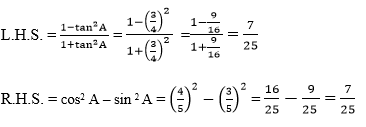Since, both the LHS and RHS = 7/25

R.H.S. =L.H.S.

Hence, (1-tanA)/(1+tan2 A) = cos2 A – sin A  is proved

9. In triangle ABC, right-angled at B, if tan A = 1/√3 find the value of:

(i) sin A cos C + cos A sin C

(ii) cos A cos C – sin A sin C

Solution:

Let ΔABC in which ∠B=90°

tan A = BC/AB = 1/√3

Let BC = 1k and AB = √3 k,

Where k is the positive real number of the problem

By Pythagoras theorem in ΔABC we get:

AC2=AB2+BC2

AC2=(√3 k)2+(k)2

AC2=3k2+k2

AC2=4k2

AC = 2k

Now find the values of cos A, Sin A

Sin A = BC/AC = 1/2

Cos A = AB/AC = √3/2

Then find the values of cos C and sin C

Sin C = AB/AC = 3/2

Cos C = BC/AC = 1/2

Now, substitute the values in the given problem

(i) sin A cos C + cos A sin C = (1/2) ×(1/2 )+ √3/2 ×√3/2 = 1/4 + 3/4 = 1

(ii) cos A cos C – sin A sin C = (3/2 )(1/2) – (1/2) (3/2 ) = 0

10. In ∆ PQR, right-angled at Q, PR + QR = 25 cm and PQ = 5 cm. Determine the values of sin P, cos P and tan P

Solution:

In a given triangle PQR, right angled at Q, the following measures are

PQ = 5 cm

PR + QR = 25 cm

Now let us assume, QR = x

PR = 25-QR

PR = 25- x

According to the Pythagorean Theorem,

PR2 = PQ2 + QR2

Substitute the value of PR as x

(25- x) 2 = 5+ x2

252 + x2 – 50x = 25 + x2

625 + x2-50x -25 – x= 0

-50x = -600

x= -600/-50

x = 12 = QR

Now, find the value of PR

PR = 25- QR

Substitute the value of QR

PR = 25-12

PR = 13

Now, substitute the value to the given problem

(1) sin p = Opposite Side/Hypotenuse = QR/PR = 12/13

(2) Cos p = Adjacent Side/Hypotenuse = PQ/PR = 5/13

(3) tan p =Opposite Side/Adjacent side = QR/PQ = 12/5

11. State whether the following are true or false. Justify your answer.

(i) The value of tan A is always less than 1.

(ii) sec A = 12/5 for some value of angle A.

(iii)cos A is the abbreviation used for the cosecant of angle A.

(iv) cot A is the product of cot and A.

(v) sin θ = 4/3 for some angle θ.

Solution:

(i) The value of tan A is always less than 1.

Proof: In ΔMNC in which ∠N = 90∘,

MN = 3, NC = 4 and MC = 5

Value of tan M = 4/3 which is greater than.

The triangle can be formed with sides equal to 3, 4 and hypotenuse = 5 as it will follow the Pythagoras theorem.

MC2=MN2+NC2

52=32+42

25=9+16

25 = 25

(ii) sec A = 12/5 for some value of angle A

Justification: Let a ΔMNC in which ∠N = 90º,

MC=12k and MB=5k, where k is a positive real number.

By Pythagoras theorem we get,

MC2=MN2+NC2

(12k)2=(5k)2+NC2

NC2+25k2=144k2

NC2=119k2

Such a triangle is possible as it will follow the Pythagoras theorem.

(iii) cos A is the abbreviation used for the cosecant of angle A.

Justification: Abbreviation used for cosecant of angle M is cosec M. cos M is the abbreviation used for cosine of angle M.

(iv) cot A is the product of cot and A.

Justification: cot M is not the product of cot and M. It is the cotangent of ∠M.

(v) sin θ = 4/3 for some angle θ.

Justification: sin θ = Height/Hypotenuse

We know that in a right angled triangle, Hypotenuse is the longest side.

∴ sin θ will always less than 1 and it can never be 4/3 for any value of θ.

Exercise 8.2 Page: 187

1. Evaluate the following:

(i) sin 60° cos 30° + sin 30° cos 60°

(ii) 2 tan2 45° + cos2 30° – sin2 60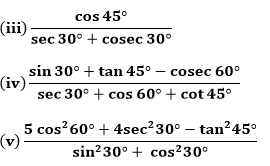Solution:

(i) sin 60° cos 30° + sin 30° cos 60°

First, find the values of the given trigonometric ratios

sin 30° = 1/2

cos 30° = √3/2

sin 60° = 3/2

cos 60°= 1/2

Now, substitute the values in the given problem

sin 60° cos 30° + sin 30° cos 60° = √3/2 ×√3/2 + (1/2) ×(1/2 ) = 3/4+1/4 = 4/4 =

(ii) 2 tan2 45° + cos2 30° – sin2 60

We know that, the values of the trigonometric ratios are:

sin 60° = √3/2

cos 30° = √3/2

tan 45° = 1

Substitute the values in the given problem

2 tan2 45° + cos2 30° – sin2 60 = 2(1)+ (√3/2)2-(√3/2)2

2 tan2 45° + cos2 30° – sin2 60 = 2 + 0

2 tan2 45° + cos2 30° – sin2 60 = 2

(iii) cos 45°/(sec 30°+cosec 30°)

We know that,

cos 45° = 1/√2

sec 30° = 2/√3

cosec 30° = 2

Substitute the values, we get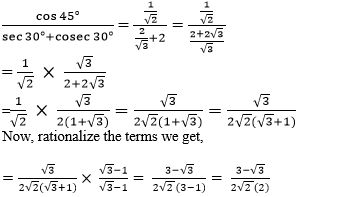Now, multiply both the numerator and denominator by √2 , we get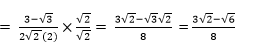Therefore, cos 45°/(sec 30°+cosec 30°) = (3√2 – √6)/8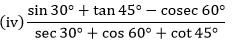We know that,

sin 30° = 1/2

tan 45° = 1

cosec 60° = 2/√3

sec 30° = 2/√3

cos 60° = 1/2

cot 45° = 1

Substitute the values in the given problem, we get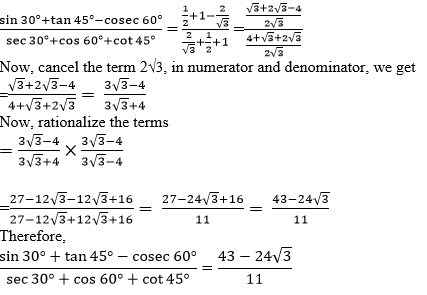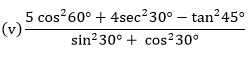We know that,

cos 60° = 1/2

sec 30° = 2/√3

tan 45° = 1

sin 30° = 1/2

cos 30° = √3/2

Now, substitute the values in the given problem, we get

(5cos260° + 4sec230° – tan245°)/(sin30° + cos30°)

= 5(1/2)2+4(2/√3)2-12/(1/2)2+(√3/2)2

= (5/4+16/3-1)/(1/4+3/4)

= (15+64-12)/12/(4/4)

= 67/12

2. Choose the correct option and justify your choice :
(i) 2tan 30°/1+tan230° =
(A) sin 60°            (B) cos 60°          (C) tan 60°            (D) sin 30°
(ii) 1-tan245°/1+tan245° =
(A) tan 90°            (B) 1                    (C) sin 45°            (D) 0
(iii)  sin 2A = 2 sin A is true when A =
(A) 0°                   (B) 30°                  (C) 45°                 (D) 60°

(iv) 2tan30°/1-tan230° =
(A) cos 60°          (B) sin 60°             (C) tan 60°           (D) sin 30°

Solution:

(i) (A) is correct.

Substitute the of tan 30° in the given equation

tan 30° = 1/√3

2tan 30°/1+tan230° = 2(1/√3)/1+(1/√3)2

= (2/√3)/(1+1/3) = (2/√3)/(4/3)

= 6/4√3 = √3/2 = sin 60°

The obtained solution is equivalent to the trigonometric ratio sin 60°

(ii) (D) is correct.

Substitute the of tan 45° in the given equation

tan 45° = 1

1-tan245°/1+tan245° = (1-12)/(1+12)

= 0/2 = 0

The solution of the above equation is 0.

(iii) (A) is correct.

To find the value of A, substitute the degree given in the options one by one

sin 2A = 2 sin A is true when A = 0°

As sin 2A = sin 0° = 0

2 sin A = 2 sin 0° = 2 × 0 = 0

or,

Apply the sin 2A formula, to find the degree value

sin 2A = 2sin A cos A

⇒2sin A cos A = 2 sin A

⇒ 2cos A = 2 ⇒ cos A = 1

Now, we have to check, to get the solution as 1, which degree value has to be applied.

When 0 degree is applied to cos value, i.e., cos 0 =1

Therefore, ⇒ A = 0°

(iv) (C) is correct.

Substitute the of tan 30° in the given equation

tan 30° = 1/√3

2tan30°/1-tan230° =  2(1/√3)/1-(1/√3)2

= (2/√3)/(1-1/3) = (2/√3)/(2/3) = √3 = tan 60°

The value of the given equation is equivalent to tan 60°.

3. If tan (A + B) = √3 and tan (A – B) = 1/√3 ,0° < A + B ≤ 90°; A > B, find A and B.

Solution:

tan (A + B) = √3

Since √3 = tan 60°

Now substitute the degree value

⇒ tan (A + B) = tan 60°

(A + B) = 60° … (i)

The above equation is assumed as equation (i)

tan (A – B) = 1/√3

Since 1/√3 = tan 30°

Now substitute the degree value

⇒ tan (A – B) = tan 30°

(A – B) = 30° … equation (ii)

Now add the equation (i) and (ii), we get

A + B + A – B = 60° + 30°

Cancel the terms B

2A = 90°

A= 45°

Now, substitute the value of A in equation (i) to find the value of B

45° + B = 60°

B = 60° – 45°

B = 15°

Therefore A = 45° and B = 15°

4. State whether the following are true or false. Justify your answer.

(i) sin (A + B) = sin A + sin B.

(ii) The value of sin θ increases as θ increases.

(iii) The value of cos θ increases as θ increases.

(iv) sin θ = cos θ for all values of θ.

(v) cot A is not defined for A = 0°.

Solution:

(i) False.

Justification:

Let us take A = 30° and B = 60°, then

Substitute the values in the sin (A + B) formula, we get

sin (A + B) = sin (30° + 60°) = sin 90° = 1 and,

sin A + sin B = sin 30° + sin 60°

= 1/2 + √3/2 = 1+√3/2

Since the values obtained are not equal, the solution is false.

(ii) True.

Justification:

According to the values obtained as per the unit circle, the values of sin are:

sin 0° = 0

sin 30° = 1/2

sin 45° = 1/√2

sin 60° = √3/2

sin 90° = 1

Thus the value of sin θ increases as θ increases. Hence, the statement is true

(iii) False.

According to the values obtained as per the unit circle, the values of cos are:

cos 0° = 1

cos 30° = √3/2

cos 45° = 1/√2

cos 60° = 1/2

cos 90° = 0

Thus, the value of cos θ decreases as θ increases. So, the statement given above is false.

(iv) False

sin θ = cos θ, when a right triangle has 2 angles of (π/4). Therefore, the above statement is false.

(v) True.

Since cot function is the reciprocal of the tan function, it is also written as:

cot A = cos A/sin A

Now substitute A = 0°

cot 0° = cos 0°/sin 0° = 1/0 = undefined.

Hence, it is true

Exercise 8.3 Page: 189

1. Evaluate :

(i) sin 18°/cos 72°

(ii) tan 26°/cot 64°

(iii)  cos 48° – sin 42°

(iv)  cosec 31° – sec 59°

Solution:

(i) sin 18°/cos 72°

To simplify this, convert the sin function into cos function

We know that, 18° is written as 90° – 18°, which is equal to the cos 72°.

= sin (90° – 18°) /cos 72°

Substitute the value, to simplify this equation

= cos 72° /cos 72° = 1

(ii) tan 26°/cot 64°

To simplify this, convert the tan function into cot function

We know that, 26° is written as 90° – 36°, which is equal to the cot 64°.

= tan (90° – 36°)/cot 64°

Substitute the value, to simplify this equation

= cot 64°/cot 64° = 1

(iii) cos 48° – sin 42°

To simplify this, convert the cos function into sin function

We know that, 48° is written as 90° – 42°, which is equal to the sin 42°.

= cos (90° – 42°) – sin 42°

Substitute the value, to simplify this equation

= sin 42° – sin 42° = 0

(iv) cosec 31° – sec 59°

To simplify this, convert the cosec function into sec function

We know that, 31° is written as 90° – 59°, which is equal to the sec 59°

= cosec (90° – 59°) – sec 59°

Substitute the value, to simplify this equation

= sec 59° – sec 59° = 0

2.  Show that:

(i) tan 48° tan 23° tan 42° tan 67° = 1

(ii) cos 38° cos 52° – sin 38° sin 52° = 0

Solution:

(i) tan 48° tan 23° tan 42° tan 67°

Simplify the given problem by converting some of the tan functions to the cot functions

We know that, tan 48° = tan (90° – 42°) = cot 42°

tan 23° = tan (90° – 67°) = cot 67°

= tan (90° – 42°) tan (90° – 67°) tan 42° tan 67°

Substitute the values

= cot 42° cot 67° tan 42° tan 67°

= (cot 42° tan 42°) (cot 67° tan 67°) = 1×1 = 1

(ii) cos 38° cos 52° – sin 38° sin 52°

Simplify the given problem by converting some of the cos functions to the sin functions

We know that,

cos 38° = cos (90° – 52°) = sin 52°

cos 52°= cos (90°-38°) = sin 38°

= cos (90° – 52°) cos (90°-38°) – sin 38° sin 52°

Substitute the values

= sin 52° sin 38° – sin 38° sin 52° = 0

3. If tan 2A = cot (A – 18°), where 2A is an acute angle, find the value of A.

Solution:

tan 2A = cot (A- 18°)

We know that tan 2A = cot (90° – 2A)

Substitute the above equation in the given problem

⇒ cot (90° – 2A) = cot (A -18°)

Now, equate the angles,

⇒ 90° – 2A = A- 18° ⇒ 108° = 3A

A = 108° / 3

Therefore, the value of A = 36°

4.  If tan A = cot B, prove that A + B = 90°.

Solution:

tan A = cot B

We know that cot B = tan (90° – B)

To prove A + B = 90°, substitute the above equation in the given problem

tan A = tan (90° – B)

A = 90° – B

A + B = 90°

Hence Proved.

5. If sec 4A = cosec (A – 20°), where 4A is an acute angle, find the value of A.

Solution:

sec 4A = cosec (A – 20°)

We know that sec 4A = cosec (90° – 4A)

To find the value of A, substitute the above equation in the given problem

cosec (90° – 4A) = cosec (A – 20°)

Now, equate the angles

90° – 4A= A- 20°

110° = 5A

A = 110°/ 5 = 22°

Therefore, the value of A = 22°

6. If A, B and C are interior angles of a triangle ABC, then show that

sin (B+C/2) = cos A/2

Solution:

We know that, for a given triangle, sum of all the interior angles of a triangle is equal to 180°

A + B + C = 180° ….(1)

To find the value of (B+ C)/2, simplify the equation (1)

⇒ B + C = 180° – A

⇒ (B+C)/2 = (180°-A)/2

⇒ (B+C)/2 = (90°-A/2)

Now, multiply both sides by sin functions, we get

⇒ sin (B+C)/2 = sin (90°-A/2)

Since sin (90°-A/2) = cos A/2, the above equation is equal to

sin (B+C)/2 = cos A/2

Hence proved.

7. Express sin 67° + cos 75° in terms of trigonometric ratios of angles between 0° and 45°.

Solution:

Given:

sin 67° + cos 75°

In term of sin as cos function and cos as sin function, it can be written as follows

sin 67° = sin (90° – 23°)

cos 75° = cos (90° – 15°)

So, sin 67° + cos 75° = sin (90° – 23°) + cos (90° – 15°)

Now, simplify the above equation

= cos 23° + sin 15°

Therefore, sin 67° + cos 75° is also expressed as cos 23° + sin 15°

Exercise 8.4 Page: 193

1. Express the trigonometric ratios sin A, sec A and tan A in terms of cot A.

Solution:

To convert the given trigonometric ratios in terms of cot functions, use trigonometric formulas

We know that,

cosec2A – cot2A = 1

cosec2A = 1 + cot2A

Since cosec function is the inverse of sin function, it is written as

1/sin2A = 1 + cot2A

Now, rearrange the terms, it becomes

sin2A = 1/(1+cot2A)

Now, take square roots on both sides, we get

sin A = ±1/(√(1+cot2A)

The above equation defines the sin function in terms of cot function

Now, to express sec function in terms of cot function, use this formula

sin2A = 1/ (1+cot2A)

Now, represent the sin function as cos function

1 – cos2A = 1/ (1+cot2A)

Rearrange the terms,

cos2A = 1 – 1/(1+cot2A)

⇒cos2A = (1-1+cot2A)/(1+cot2A)

Since sec function is the inverse of cos function,

⇒ 1/sec2A = cot2A/(1+cot2A)

Take the reciprocal and square roots on both sides, we get

⇒ sec A = ±√ (1+cot2A)/cotA

Now, to express tan function in terms of cot function

tan A = sin A/cos A and cot A = cos A/sin A

Since cot function is the inverse of tan function, it is rewritten as

tan A = 1/cot A

2. Write all the other trigonometric ratios of ∠A in terms of sec A.

Solution:

Cos A function in terms of sec A:

sec A = 1/cos A

⇒ cos A = 1/sec A

sec A function in terms of sec A:

cos2A + sin2A = 1

Rearrange the terms

sin2A = 1 – cos2A

sin2A = 1 – (1/sec2A)

sin2A = (sec2A-1)/sec2A

sin A = ± √(sec2A-1)/sec A

cosec A function in terms of sec A:

sin A = 1/cosec A

⇒cosec A = 1/sin A

cosec A = ± sec A/√(sec2A-1)

Now, tan A function in terms of sec A:

sec2A – tan2A = 1

Rearrange the terms

⇒ tan2A = sec2A – 1

tan A = √(sec2A – 1)

cot A function in terms of sec A:

tan A = 1/cot A

⇒ cot A = 1/tan A

cot A = ±1/√(sec2A – 1)

3. Evaluate:

(i) (sin263° + sin227°)/(cos217° + cos273°)
(ii)  sin 25° cos 65° + cos 25° sin 65°

Solution:

(i) (sin263° + sin227°)/(cos217° + cos273°)

To simplify this, convert some of the sin functions into cos functions and cos function into sin function and it becomes,

= [sin2(90°-27°) + sin227°] / [cos2(90°-73°) + cos273°)]

= (cos227° + sin227°)/(sin227° + cos273°)

= 1/1 =1                       (since sin2A + cos2A = 1)

Therefore, (sin263° + sin227°)/(cos217° + cos273°) = 1

(ii) sin 25° cos 65° + cos 25° sin 65°

To simplify this, convert some of the sin functions into cos functions and cos function into sin function and it becomes,

= sin(90°-25°) cos 65° + cos (90°-65°) sin 65°

= cos 65° cos 65° + sin 65° sin 65°

= cos265° + sin265° = 1 (since sin2A + cos2A = 1)

Therefore, sin 25° cos 65° + cos 25° sin 65° = 1

4. Choose the correct option. Justify your choice.
(i) 9 sec2A – 9 tan2A =
(A) 1                 (B) 9              (C) 8                (D) 0
(ii) (1 + tan θ + sec θ) (1 + cot θ – cosec θ)
(A) 0                 (B) 1              (C) 2                (D) – 1
(iii) (sec A + tan A) (1 – sin A) =
(A) sec A           (B) sin A        (C) cosec A      (D) cos A

(iv) 1+tan2A/1+cot2A =

(A) secA                 (B) -1              (C) cot2A                (D) tan2A

Solution:

(i) (B) is correct.

Justification:

Take 9 outside, and it becomes

9 sec2A – 9 tan2A

= 9 (sec2A – tan2A)

= 9×1 = 9             (∵ sec2 A – tan2 A = 1)

Therefore, 9 sec2A – 9 tan2A = 9

(ii) (C) is correct

Justification:

(1 + tan θ + sec θ) (1 + cot θ – cosec θ)

We know that, tan θ = sin θ/cos θ

sec θ = 1/ cos θ

cot θ = cos θ/sin θ

cosec θ = 1/sin θ

Now, substitute the above values in the given problem, we get

= (1 + sin θ/cos θ + 1/ cos θ) (1 + cos θ/sin θ – 1/sin θ)

Simplify the above equation,

= (cos θ +sin θ+1)/cos θ × (sin θ+cos θ-1)/sin θ

= (cos θ+sin θ)2-12/(cos θ sin θ)

= (cos2θ + sin2θ + 2cos θ sin θ -1)/(cos θ sin θ)

= (1+ 2cos θ sin θ -1)/(cos θ sin θ) (Since cos2θ + sin2θ = 1)

= (2cos θ sin θ)/(cos θ sin θ) = 2

Therefore, (1 + tan θ + sec θ) (1 + cot θ – cosec θ) =2

(iii) (D) is correct.

Justification:

We know that,

Sec A= 1/cos A

Tan A = sin A / cos A

Now, substitute the above values in the given problem, we get

(secA + tanA) (1 – sinA)

= (1/cos A + sin A/cos A) (1 – sinA)

= (1+sin A/cos A) (1 – sinA)

= (1 – sin2A)/cos A

= cos2A/cos A = cos A

Therefore, (secA + tanA) (1 – sinA) = cos A

(iv) (D) is correct.

Justification:

We know that,

tan2A =1/cot2A

Now, substitute this in the given problem, we get

1+tan2A/1+cot2A

= (1+1/cot2A)/1+cot2A

= (cot2A+1/cot2A)×(1/1+cot2A)

= 1/cot2A = tan2A

So, 1+tan2A/1+cot2A = tan2A

5. Prove the following identities, where the angles involved are acute angles for which the
expressions are defined.

(i) (cosec θ – cot θ)= (1-cos θ)/(1+cos θ)

(ii) cos A/(1+sin A) + (1+sin A)/cos A = 2 sec A

(iii) tan θ/(1-cot θ) + cot θ/(1-tan θ) = 1 + sec θ cosec θ

[Hint : Write the expression in terms of sin θ and cos θ]

(iv) (1 + sec A)/sec A = sin2A/(1-cos A)

[Hint : Simplify LHS and RHS separately]

(v) ( cos A–sin A+1)/( cos A +sin A–1) = cosec A + cot A, using the identity cosec2A = 1+cot2A.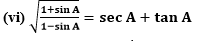(vii) (sin θ – 2sin3θ)/(2cos3θ-cos θ) = tan θ
(viii) (sin A + cosec A)+ (cos A + sec A)2 = 7+tan2A+cot2A
(ix) (cosec A – sin A)(sec A – cos A) = 1/(tan A+cotA)
[Hint : Simplify LHS and RHS separately]
(x) (1+tan2A/1+cot2A) = (1-tan A/1-cot A)2 = tan2A

Solution:

(i) (cosec θ – cot θ)= (1-cos θ)/(1+cos θ)

To prove this, first take the Left-Hand side (L.H.S) of the given equation, to prove the Right Hand Side (R.H.S)

L.H.S. = (cosec θ – cot θ)2

The above equation is in the form of (a-b)2, and expand it

Since (a-b)2 = a2 + b2 – 2ab

Here a = cosec θ and b = cot θ

= (cosec2θ + cot2θ – 2cosec θ cot θ)

Now, apply the corresponding inverse functions and equivalent ratios to simplify

= (1/sin2θ + cos2θ/sin2θ – 2cos θ/sin2θ)

= (1 + cos2θ – 2cos θ)/(1 – cos2θ)

= (1-cos θ)2/(1 – cosθ)(1+cos θ)

= (1-cos θ)/(1+cos θ) = R.H.S.

Therefore, (cosec θ – cot θ)= (1-cos θ)/(1+cos θ)

Hence proved.

(ii)  (cos A/(1+sin A)) + ((1+sin A)/cos A) = 2 sec A

Now, take the L.H.S of the given equation.

L.H.S. = (cos A/(1+sin A)) + ((1+sin A)/cos A)

= [cos2A + (1+sin A)2]/(1+sin A)cos A

= (cos2A + sin2A + 1 + 2sin A)/(1+sin A) cos A

Since cos2A + sin2A = 1, we can write it as

= (1 + 1 + 2sin A)/(1+sin A) cos A

= (2+ 2sin A)/(1+sin A)cos A

= 2(1+sin A)/(1+sin A)cos A

= 2/cos A = 2 sec A = R.H.S.

L.H.S. = R.H.S.

(cos A/(1+sin A)) + ((1+sin A)/cos A) = 2 sec A

Hence proved.

(iii) tan θ/(1-cot θ) + cot θ/(1-tan θ) = 1 + sec θ cosec θ

L.H.S. = tan θ/(1-cot θ) + cot θ/(1-tan θ)

We know that tan θ =sin θ/cos θ

cot θ = cos θ/sin θ

Now, substitute it in the given equation, to convert it in a simplified form

= [(sin θ/cos θ)/1-(cos θ/sin θ)] + [(cos θ/sin θ)/1-(sin θ/cos θ)]

= [(sin θ/cos θ)/(sin θ-cos θ)/sin θ] + [(cos θ/sin θ)/(cos θ-sin θ)/cos θ]

= sin2θ/[cos θ(sin θ-cos θ)] + cos2θ/[sin θ(cos θ-sin θ)]

= sin2θ/[cos θ(sin θ-cos θ)] – cos2θ/[sin θ(sin θ-cos θ)]

= 1/(sin θ-cos θ) [(sin2θ/cos θ) – (cos2θ/sin θ)]

= 1/(sin θ-cos θ) × [(sin3θ – cos3θ)/sin θ cos θ]

= [(sin θ-cos θ)(sin2θ+cos2θ+sin θ cos θ)]/[(sin θ-cos θ)sin θ cos θ]

= (1 + sin θ cos θ)/sin θ cos θ

= 1/sin θ cos θ + 1

= 1 + sec θ cosec θ = R.H.S.

Therefore, L.H.S. = R.H.S.

Hence proved

(iv)  (1 + sec A)/sec A = sin2A/(1-cos A)

First find the simplified form of L.H.S

L.H.S. = (1 + sec A)/sec A

Since secant function is the inverse function of cos function and it is written as

= (1 + 1/cos A)/1/cos A

= (cos A + 1)/cos A/1/cos A

Therefore, (1 + sec A)/sec A = cos A + 1

R.H.S. = sin2A/(1-cos A)

We know that sin2A = (1 – cos2A), we get

= (1 – cos2A)/(1-cos A)

= (1-cos A)(1+cos A)/(1-cos A)

Therefore, sin2A/(1-cos A)= cos A + 1

L.H.S. = R.H.S.

Hence proved

(v) (cos A–sin A+1)/(cos A+sin A–1) = cosec A + cot A, using the identity cosec2A = 1+cot2A.

With the help of identity function, cosec2A = 1+cot2A, let us prove the above equation.

L.H.S. = (cos A–sin A+1)/(cos A+sin A–1)

Divide the numerator and denominator by sin A, we get

= (cos A–sin A+1)/sin A/(cos A+sin A–1)/sin A

We know that cos A/sin A = cot A and 1/sin A = cosec A

= (cot A – 1 + cosec A)/(cot A+ 1 – cosec A)

= (cot A – cosec2A + cot2A + cosec A)/(cot A+ 1 – cosec A) (using cosec2A – cot2A = 1

= [(cot A + cosec A) – (cosec2A – cot2A)]/(cot A+ 1 – cosec A)

= [(cot A + cosec A) – (cosec A + cot A)(cosec A – cot A)]/(1 – cosec A + cot A)

=  (cot A + cosec A)(1 – cosec A + cot A)/(1 – cosec A + cot A)

=  cot A + cosec A = R.H.S.

Therefore, (cos A–sin A+1)/(cos A+sin A–1) = cosec A + cot A

Hence Proved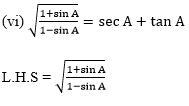First divide the numerator and denominator of L.H.S. by cos A,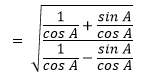We know that 1/cos A = sec A and sin A/ cos A = tan A and it becomes,

= √(sec A+ tan A)/(sec A-tan A)

Now using rationalization, we get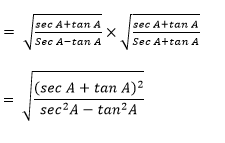= (sec A + tan A)/1

= sec A + tan A = R.H.S

Hence proved

(vii) (sin θ – 2sin3θ)/(2cos3θ-cos θ) = tan θ

L.H.S. = (sin θ – 2sin3θ)/(2cos3θ – cos θ)

Take sin θ as in numerator and cos θ in denominator as outside, it becomes

= [sin θ(1 – 2sin2θ)]/[cos θ(2cos2θ- 1)]

We know that sin2θ = 1-cos2θ

= sin θ[1 – 2(1-cos2θ)]/[cos θ(2cos2θ -1)]

= [sin θ(2cos2θ -1)]/[cos θ(2cos2θ -1)]

= tan θ = R.H.S.

Hence proved

(viii) (sin A + cosec A)+ (cos A + sec A)2 = 7+tan2A+cot2A

L.H.S. = (sin A + cosec A)+ (cos A + sec A)2

It is of the form (a+b)2, expand it

(a+b)2 =a2 + b2 +2ab

= (sin2A + cosec2A + 2 sin A cosec A) + (cos2A + sec2A + 2 cos A sec A)

= (sin2A + cos2A) + 2 sin A(1/sin A) + 2 cos A(1/cos A) + 1 + tan2A + 1 + cot2A

= 1 + 2 + 2 + 2 + tan2A + cot2A

= 7+tan2A+cot2A = R.H.S.

Therefore, (sin A + cosec A)+ (cos A + sec A)2 = 7+tan2A+cot2A

Hence proved.

(ix) (cosec A – sin A)(sec A – cos A) = 1/(tan A+cotA)

First, find the simplified form of L.H.S

L.H.S. = (cosec A – sin A)(sec A – cos A)

Now, substitute the inverse and equivalent trigonometric ratio forms

= (1/sin A – sin A)(1/cos A – cos A)

= [(1-sin2A)/sin A][(1-cos2A)/cos A]

= (cos2A/sin A)×(sin2A/cos A)

= cos A sin A

Now, simplify the R.H.S

R.H.S. = 1/(tan A+cotA)

= 1/(sin A/cos A +cos A/sin A)

= 1/[(sin2A+cos2A)/sin A cos A]

= cos A sin A

L.H.S. = R.H.S.

(cosec A – sin A)(sec A – cos A) = 1/(tan A+cotA)

Hence proved

(x)  (1+tan2A/1+cot2A) = (1-tan A/1-cot A)2 = tan2A

L.H.S. = (1+tan2A/1+cot2A)

Since cot function is the inverse of tan function,

= (1+tan2A/1+1/tan2A)

= 1+tan2A/[(1+tan2A)/tan2A]

Now cancel the 1+tan2A terms, we get

= tan2A

(1+tan2A/1+cot2A) = tan2A

Similarly,

(1-tan A/1-cot A)2 = tan2A

Hence proved

### Comprehensive List Of Study Materials